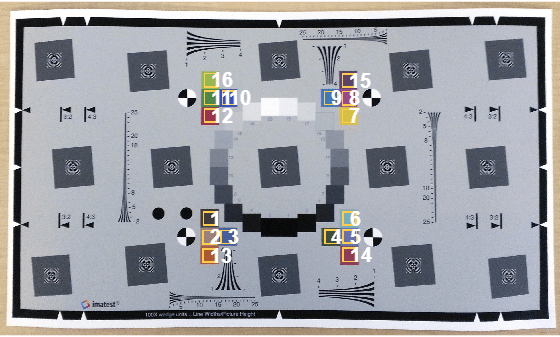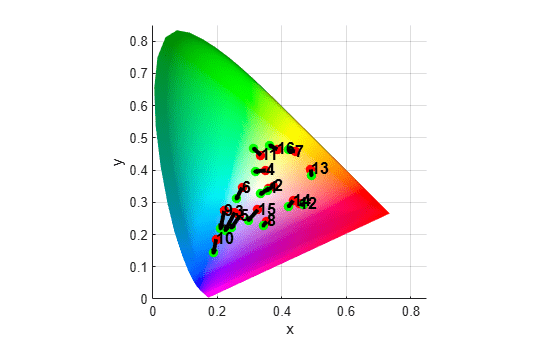Documentation

# plotChromaticity

Plot color reproduction on chromaticity diagram

## Syntax

``plotChromaticity(colorTable)``
``plotChromaticity(colorTable,Name,Value)``
``plotChromaticity``
``plotChromaticity(Name,Value)``

## Description

example

````plotChromaticity(colorTable)` plots on a chromaticity diagram the measured and reference colors, `colorTable`, for color patch regions of interest (ROIs) in a test chart.```
````plotChromaticity(colorTable,Name,Value)` plots measured and reference colors with additional parameters to control aspects of the display.```
````plotChromaticity` plots an empty chromaticity diagram.```
````plotChromaticity(Name,Value)` plots an empty chromaticity diagram with additional parameter `'Parent'` that specifies a handle of a parent axes of the plot object.```

## Examples

collapse all

This example shows how to display the chromaticity diagram from measurements of color accuracy on an Imatest® eSFR chart.

Read an image of an eSFR chart into the workspace.

`I = imread('eSFRTestImage.jpg');`

Create an `esfrChart` object. Display the chart, highlighting the 16 color patches.

```chart = esfrChart(I); displayChart(chart,'displayEdgeROIs',false, ... 'displayGrayROIs',false,'displayRegistrationPoints',false)```Measure the color in all color patch ROIs.

`colorTable = measureColor(chart);`

Plot the measured and reference colors in the CIE 1976 L*a*b* color space on a chromaticity diagram. Red circles indicate the reference color and green circles indicate the measured color of each color patch. The chromaticity diagram does not portray the brightness of color.

```figure plotChromaticity(colorTable)```## Input Arguments

collapse all

Color values in each color patch, specified as an m-by-8 color table, where m is the number of patches. The eight columns represent these variables:

VariableDescription
`ROI`Index of the sampled ROI. The value of `ROI` is an integer in the range [1, 16]. The indices match the ROI numbers displayed by `displayChart`.
`Measured_R`

Mean value of red channel pixels in an ROI. `Measured_R` is a scalar of the same data type as `chart.Image`, which can be of type `single`, `double`, `uint8`, or `uint16`.

`Measured_G`

Mean value of green channel pixels in an ROI. `Measured_G` is a scalar of the same data type as `chart.Image`.

`Measured_B`

Mean value of blue channel pixels in an ROI. `Measured_B` is a scalar of the same data type as `chart.Image`.

`Reference_L`

Reference L* value corresponding to the ROI. `Reference_L` is a scalar of type `double`.

`Reference_a`

Reference a* value corresponding to the ROI. `Reference_a` is a scalar of type `double`.

`Reference_b`

Reference b* value corresponding to the ROI. `Reference_b` is a scalar of type `double`.

`Delta_E`Euclidean color distance between the measured and reference color values, as outlined in CIE 1976.

To obtain a color table, use the `measureColor` function.

### Name-Value Pair Arguments

Specify optional comma-separated pairs of `Name,Value` arguments. `Name` is the argument name and `Value` is the corresponding value. `Name` must appear inside quotes. You can specify several name and value pair arguments in any order as `Name1,Value1,...,NameN,ValueN`.

Example: `plotChromaticity(myColorTable,'displayROIIndex',false)` turns off the display of the ROI indices on the chromaticity diagram.

Display ROI index labels, specified as the comma-separated pair consisting of `'displayROIIndex'` and `true` or `false`. When `displayROIIndex` is `true`, then `plotChromaticity` overlays color patch ROI index labels on the chromaticity diagram. The indices match the ROI numbers displayed by `displayChart`.

Data Types: `logical`

Axes handle of the displayed image object, specified as the comma-separated pair consisting of `'Parent'` and an axes handle. `Parent` specifies the parent of the image object created by `plotChromaticity`.